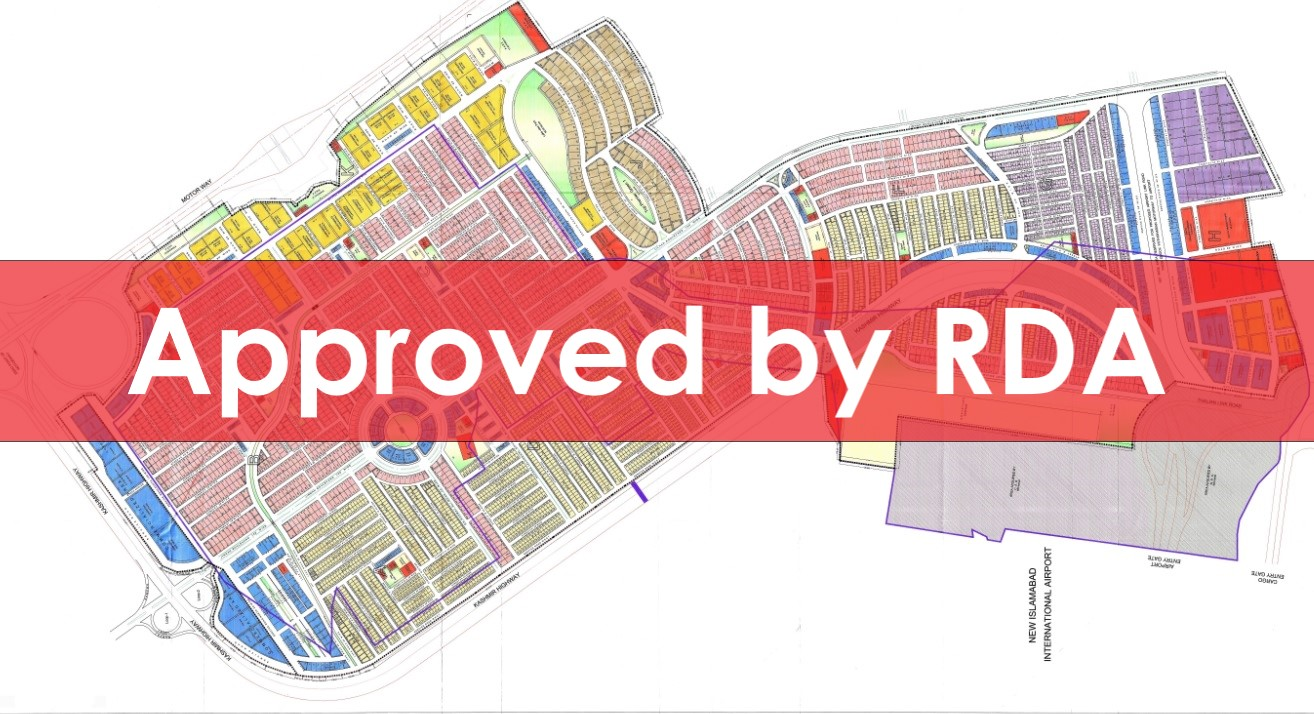# Top City-1 Islamabad Revised Master Plan Map Approved by RDATop City-1 has finally got approval of its revised master plan map from Rawalpindi Development Authority. The revised plan was already available in the market for quite some time but all it needed was approval from RDA in order to be used precisely for reference.

The revised master plan spans over 9081 kanals of land, and it includes some new sectors including G, I & J. Some blocks have been reshuffled, and redesigned, while some have expanded to include more plots.

The land use distribution in the revised plan is given as under:

• Residential = 4287.47 kanals
• Commercial = 443.40 kanals
• Green Spaces = 620.76 kanals
• Grave Yard = 177.36 kanals
• Public Building = 384.91 kanals
• Commercialized Area (High Rise Buildings) = 152 Kanals
• Grave Yard (Mumtaz City) = 61

The number of plots for each plot size is given as under:

• 100×180 = 51 plots
• 75×120 = 170 plots
• 50×90 = 2419 plots
• 35×65 = 2805 plots
• 25×50 = 1039 plots
• Apartments = 46 plots

The number of plots in each block is given as under:

• Block A = 839 plots
• Block B = 763 plots
• Block C = 838 plots
• Block D = 1453 plots
• Block E = 545 plots
• Block F = 653 plots
• Block G = 840 plots
• Block H = 51 plots
• Block I = 288 plots
• Block J = 214 plots

Top City-1 has launched new bookings of residential plots, commercial plots, and high-rise tower plots right after the approval of its revised map. You can check the details of new bookings at the following page: